# Alley

Alley measured a meters. At the beginning and end are planted poplar. How many we must plant poplars to get the distance between the poplars 15 meters?

Result

n = (Correct answer is: a/15-1)#### Solution: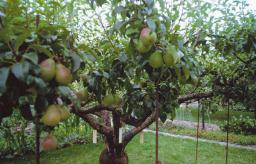We would be pleased if you find an error in the word problem, spelling mistakes, or inaccuracies and send it to us. Thank you!Tips to related online calculators
Do you want to convert length units?

## Next similar math problems:

• Trees in alleyThere are four trees in the alley between which the distances are 35m, 15m and 95m. Trees must be laid in the spaces so that the distance is the same and the maximum. How many trees will they put in and what will be the distance between them?
• Perimeter to areaCalculate the area of a circle with the perimeter 15 meters.
• Rectangular flowerbedAround the rectangular flowerbed with dimensions of 5.25 m and 3.50 m, roses should be planted at the same distance from each other so that the roses are located in each corner of the flower bed and are consumed as little as possible. How far do we plant
• Interst on savings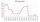The bank offers 1.6% interest. How many euros have to insert at the beginning if we received € 15 on the interest?
• FlowerbedsWhat is the smallest length of flowerbeds we have to prepare to plant seedlings at grid 20,30,25,40 cm?
• Maria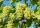Maria has decided to plant grapes in the garden behind her house. His neighbor Ben has grown grapes successfully for a long time and has given Maria advice on how to plant vines. Ben told her to plant them 3 meters apart in rows that are also 3 meters apa
• Cube basicsHow long is the edge length of a cube with volume 15 m3?
• 15 numberWhat number is smaller (greater) by 15 than its half?
• Street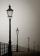Straight streets is long x = 475 meters. At the beginning and at the end of the street are poles. The poles are spaced 25 m. How many poles are on the street?
• Jumps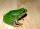At least how many jumps must do frog to overcome the distance of 7 meters? Jump of frog is 18 cm long.
• Two rulers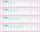We have two rulers. Scale interval on first rulers are a spaced 1 cm and on second spaced 15 mm. Rulers are attached to each other so that they match initial divider commas. What next dividers commas coincide? Find at least three cases.
• Bus linesBuses connections are started from bus stop on its regular circuit as follows: No. 27 bus every 27 minutes and No.18 every half hour. What time started this two bus lines run if the bus stop met at 10:15 am.?
• Steps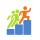Peter walked a distance of 60 m 102 steps. How many steps must he take to walk 150 m?
• Lcm of three numbersWhat is the Lcm of 120 15 and 5
• Four poplarsFour poplars are growing along the way. The distances between them are 35 m, 14 m, and 91 m. At least how many poplars need to be dropped to create the same spacing between the trees? How many meters will it be?
• Chocholate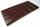Table of chocolate is divided into squares on its surface. Lengthwise has 15 squares and widthwise 19 squares. We must chocolate broke into individual squares. How many times we have broke it to get only individual squares? It is not permitted to break se
• BasenHow many square meters of tiles we need to tile the walls and floor of the pool 15 meters long, six meters wide and two meters?deep